# Hybrid Images. Einstein-Monroe¶

### Examples - Astrophysics¶

Last edited: March 22nd 2018

## Introduction¶

Looking at the image below, who do you see? The famous physicist Albert Einstein, or the popular sex symbol of the 1950s, Marylin Monroe?

From a short distance a sharp image of Einstein can be seen, with only a blurred distortion hinting at the presence of a superimposed image. But, viewed from a distance or while squinting, the finer details of Einstein get blurred and the unmistakable face of Monroe appears! Note also that if the viewer is near-sighted, they may see Monroe at a normal viewing distance.Figure 1: Behind this great man is a beautiful woman .

The image is one of the most famous examples of a so-called hybrid image. It is created by combining two images where one is filtered with a low pass filter and the other with a high pass filter . The concept is based on the way we humans process visual input. A scheme for creating images with this optical illusion was developed by Aude Oliva of MIT and Philippe G. Schyns of University of Glasgow.

One physical explanation for this effect is the spatial resolution of our eyes, estimated by Rayleigh's criterion, $\Delta l_{min} = 1.22\lambda R/D$ . Here, $D$ is the diameter of the lens' aperture, $\lambda$ is the wavelength of the light and $R$ is the distance to the object or image. As an example, let $R=25\mathrm{cm}$ (the ideal reading distance), $D\approx4\mathrm{mm}$ (the diameter of the pupils in our eyes) and $\lambda=550\mathrm{nm}$ (middle of the visible spectrum). Then, the finest details we can resolve in the image is $\Delta l_{min} \approx$ 0.04 mm. Poorer resolution, i.e. an increased $\Delta l_{min}$, is obtained by increasing the distance to the image $R$ or by squinting and thus decreasing $D$. Hence, the finer details of Einstein cannot be resolved when $\Delta l_{min}$ increases, and the image of Monroe takes precedence.

Using the tools introduced in our notebook Image Filtering using Fourier Transform, it should be easy to analyse the concept of hybrid images. In this notebook we try to use a low pass and a high pass filter to extract extract Monroe and Einstein, respectively. Toward the end we create our own, simplified hybrid image!

We start by importing packages, setting figure parameters and defining the functions defined in the aforementioned notebook on image filtering.

In :
import matplotlib.pyplot as plt
import numpy as np
import scipy.fftpack as fft
from skimage import io # used to read images from url
import warnings
warnings.filterwarnings("ignore")

def FFT(image):
""" Compute the 2-dimensional discrete Fourier Transform of an image
and shift the zero-frequency component to the center of the spectrum.

Parameters:
image: array-like, shape (m, n), can be complex. Image being transformed.
Returns: complex ndarray, shape (m, n). Frequency spectrum.
"""
return np.fft.fftshift(np.fft.fft2(image))

def IFFT(spectrum):
""" Shift the zero-frequency components to the edges of the spectrum and
compute the inverse 2-dimensional discrete Fourier Transform of an image.

Parameters:
image: array-like, shape (m, n), can be complex. Spectrum being transformed.
Returns: complex ndarray, shape (m, n). Spacial domain.
"""
return np.fft.ifft2(np.fft.fftshift(spectrum))

def filter_spectrum(spectrum, filter_type=None, val=50):
""" Filter the spectrum of an image using a gaussian high pass or low pass filter.

Parameters:
spectrum:    array-like, shape (m, n), can be complex. Spectrum being filtered.
filter_type: string. Filter name, {'gaussian_highpass', 'gaussian_lowpass'}.
val:         float. Filter parameter.
Returns:         complex 2d-array, shape (m,n). Filtered spectrum.
"""
m, n = np.shape(spectrum)
x, y = np.meshgrid(np.arange(m), np.arange(n))
R2 = np.transpose((x - m/2)**2 + (y - n/2)**2)
if (filter_type == 'gaussian_highpass'):
return spectrum*(1 - np.exp(-val*R2))
elif (filter_type == 'gaussian_lowpass'):
return spectrum*np.exp(-val*R2)
elif (filter_type != None):
raise ValueError('%s is not a valid filter!' % filter_type)
return spectrum

def normalise(image):
""" Normalise an image such that the minimal pixel value becomes 0 and the maximum
pixel value becomes 1. Only the real value of the image is used in case of
numerical rounding errors during the Fourier Transforms.

Parameters:
image: array-like, shape (m, n), can be complex. Image being transformed.
Returns: real 2d-array(). Normalised image.
"""
image = np.real(image)
return (image - np.min(image))/(np.max(image) - np.min(image))


Let us import the Einstein-Monroe image, perform the Fourier Transform to obtain the frequency spectrum and then visualise the result.

In :
# Import image, originally downloaded from 
as_grey=True)

# Compute the frequency spectrum
spec = FFT(img)

# Plot the result
plt.subplot(121)
plt.imshow(img, cmap='gray')
plt.subplot(122)
plt.imshow(np.log(abs(spec)), cmap='gray');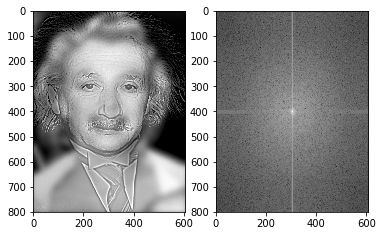Now everything is in place to filter the image! We want to filter such that only one of the two persons are visible. Keep in mind that the images obtained will have a poorer quality than the original images (why?).

## Extracting frequencies. High = Einstein, low = Monroe¶

We now use the gaussian highpass filter to exclude the low frequencies to get Einstein and the gaussian lowpass filter to exclude the high frequencies to get Monroe. After trying with different excluding values we obtain images where Einstein and Monroe can be seen seperately.

In :
# Filter spectra
filtered_spec_einstein = filter_spectrum(spec, filter_type='gaussian_highpass', val=0.001)
filtered_spec_monroe = filter_spectrum(spec, filter_type='gaussian_lowpass', val=0.01)

# Transform into filtered images
filtered_img_einstein = np.real(IFFT(filtered_spec_einstein))
filtered_img_monroe = np.real(IFFT(filtered_spec_monroe))

# Plot result
plt.subplot(121)
plt.imshow(filtered_img_einstein, cmap='gray');
plt.subplot(122)
plt.imshow(filtered_img_monroe, cmap='gray');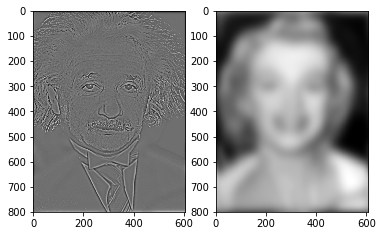## Create our own hybrid image¶

It is time to create a hybrid image! We will use an image of Leonard Nimoy as Spock  and William Shatner as James T. Kirk  from Star Trek. Note that the images should be under proper alignment for the best optical illusion effect. Some additional tips are explained in the article on hybrid images by Aude Oliva, Antonio Torralba and Philippe. G. Schyns from 2008. We start by importing the images and visualising them.

In :
spock = io.imread('https://www.numfys.net/media/notebooks/images/spock.jpg', True)
plt.subplot(121)
plt.imshow(spock, cmap='gray')
plt.subplot(122)
plt.imshow(kirk, cmap='gray');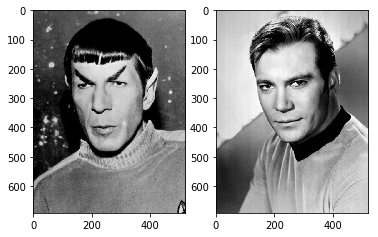Now we filter the images. Let Spock be highpass filtered and Kirk be lowpass filtered. Play around with the parameters!

In :
# Filter Spock
spock_spec = FFT(spock)
filtered_spock_spec = filter_spectrum(spock_spec, filter_type='gaussian_highpass', val=0.001)
filtered_spock = np.real(IFFT(filtered_spock_spec))

# Filter Kirk
kirk_spec = FFT(kirk)
filtered_kirk_spec = filter_spectrum(kirk_spec, filter_type='gaussian_lowpass', val=0.005)
filtered_kirk = np.real(IFFT(filtered_kirk_spec))

# Plot the result
plt.subplot(121)
plt.imshow(filtered_spock, cmap='gray')
plt.subplot(122)
plt.imshow(filtered_kirk, cmap='gray');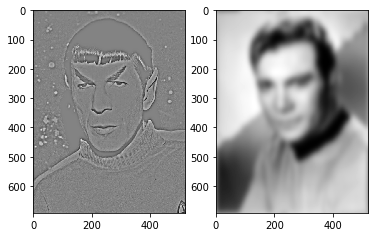We now normalise the images, add them together and plot the result. And we have our hybrid image!

In :
# Normalise the individual images
filtered_spock = normalise(filtered_spock)
filtered_kirk = normalise(filtered_kirk)

# Create the hybrid image
spock_kirk_hybrid = filtered_spock + filtered_kirk

# Plot the result
plt.imshow(spock_kirk_hybrid, cmap='gray');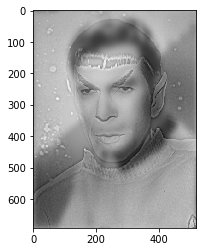## Further work and improvements¶

• How can this be done for an image with colors? Hint: how do a computer store images with colors?
• Would it be possible to add more than two images in a hybrid image?
• Are there any applications of hybrid images (e.g. commercials)?

## Resources¶

 Wikipedia. Angular resolution https://en.wikipedia.org/wiki/Angular_resolution, 10-25-106 [acquired 11-08-2016]

 Aude Oliva, Antonio Torralba, Philippe. G. Schyns. Hybrid Images, http://cvcl.mit.edu/hybrid/OlivaTorralb_Hybrid_Siggraph06.pdf, 2008 [aquired 10-29-2016]

 Wikipedia. Hybrid image, https://en.wikipedia.org/wiki/Hybrid_image, 10-26-2007 [aquired 10-28-2016]

### Pictures:¶

 Gregory T. Huang: Einstein-Monroe, https://www.newscientist.com/article/mg19325971-600-hybrid-images-now-you-see-them/?DCMP=ILC-Top5&;href=images, 03-28-2007 [aquired 10-28-2016]

 NBC television: Leonard Nimoy as Spock, https://commons.wikimedia.org/wiki/File:Leonard_Nimoy_as_Spock_1967.jpg, 1967 [aquired 10-28-2016]

 NBC television: William shatner as James T. Kirk, https://commons.wikimedia.org/wiki/File:Star_Trek_William_Shatner.JPG, 1967 [aquired 10-28-2016]